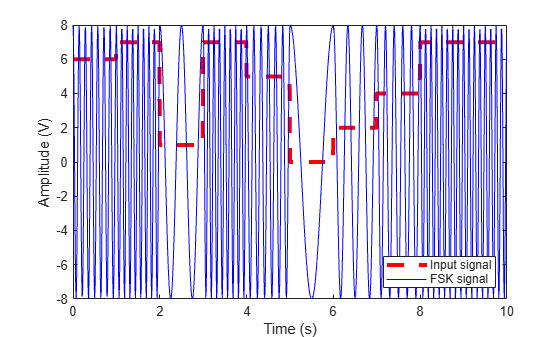Documentation

This is machine translation

Mouseover text to see original. Click the button below to return to the English version of the page.

comm.DiscreteTimeVCO

Generate variable frequency sinusoid

Description

The `DiscreteTimeVCO` (voltage-controlled oscillator) object generates a signal whose frequency shift from the quiescent frequency property is proportional to the input signal. The input signal is interpreted as a voltage.

To generate a variable frequency sinusoid:

1. Define and set up your discrete time voltage-controlled oscillator object. See Construction .

2. Call `step` to generate a variable frequency sinusoid according to the properties of `comm.DiscreteTimeVCO`. The behavior of `step` is specific to each object in the toolbox.

Note

Starting in R2016b, instead of using the `step` method to perform the operation defined by the System object™, you can call the object with arguments, as if it were a function. For example, ```y = step(obj,x)``` and `y = obj(x)` perform equivalent operations.

Construction

`H = comm.DiscreteTimeVCO` creates a discrete-time voltage-controlled oscillator (VCO) System object, `H`. This object generates a sinusoidal signal with the frequency shifted from the specified quiescent frequency to a value proportional to the input signal.

`H = comm.DiscreteTimeVCO(Name,Value)` creates a discrete-time VCO object, `H`, with each specified property set to the specified value. You can specify additional name-value pair arguments in any order as (`Name1`,`Value1`,...,`NameN`,`ValueN`).

Properties

 `OutputAmplitude` Amplitude of output signal Specify the amplitude of the output signal as a double- or single-precision, scalar value. The default is `1`. This property is tunable. `QuiescentFrequency` Frequency of output signal when input is zero Specify the quiescent frequency of the output signal in Hertz, as a double- or single-precision, real, scalar value. The default is `10`. This property is tunable. `Sensitivity` Sensitivity of frequency shift of output signal Specify the sensitivity of the output signal frequency shift to the input as a double- or single-precision, real, scalar value. The default is `1`. This value scales the input voltage and, consequently, the shift from the quiescent frequency value. The property measures Sensitivity in Hertz per volt. This property is tunable. `InitialPhase` Initial phase of output signal Specify the initial phase of the output signal, in radians, as a double or single precision, real, scalar value. The default is `0`. `SampleRate` Sample rate of input Specify the sample rate of the input, in Hertz, as a double- or single-precision, positive, scalar value. The default is `100`.

Methods

 reset Reset states of discrete-time VCO object step Generate variable frequency sinusoid
Common to All System Objects
`release`

Allow System object property value changes

Examples

expand all

Create a signal source System object™.

`reader = dsp.SignalSource;`

Generate random data and apply rectangular pulse shaping.

```reader.Signal = randi([0 7],10,1); reader.Signal = rectpulse(reader.Signal,100);```

Create a signal logger and discrete time VCO System objects.

```logger = dsp.SignalSink; discreteVCO = comm.DiscreteTimeVCO('OutputAmplitude',8,'QuiescentFrequency',1);```

Generate an FSK signal.

```while(~isDone(reader)) sig = reader(); y = discreteVCO(sig); logger(y); end oscsig = logger.Buffer;```

Plot the generated FSK signal.

```t = (0:length(oscsig)-1)'/discreteVCO.SampleRate; plot(t,reader.Signal,'--r', 'LineWidth',3) hold on plot(t,oscsig,'-b'); hold off xlabel('Time (s)') ylabel('Amplitude (V)') legend('Input Signal','FSK Signal','location','se')```Algorithms

This object implements the algorithm, inputs, and outputs as described on the Discrete-Time VCO block reference page. However, this object and the corresponding block may not generate the exact same outputs for single-precision inputs or property values due to the following differences in casting strategies and arithmetic precision issues:

• The block always casts the result of intermediate mathematical operations to the input data type. The object does not cast intermediate results and MATLAB decides the data type. The object casts the final output to the input data type.

• You can specify the `SampleRate` object property in single-precision or double-precision. The block does not allow this.

• In arithmetic operations with more than two operands with mixed data types, the result may differ depending on the order of operation. Thus, the following calculation may also contribute to the difference in the output of the block and the object:

input * sensitivity * `sampleTime`

• The block performs this calculation from left to right. However, since sensitivity * `sampleTime` is a one-time calculation, the object calculates this in the following manner:

input * (sensitivity * `sampleTime`)